Publicité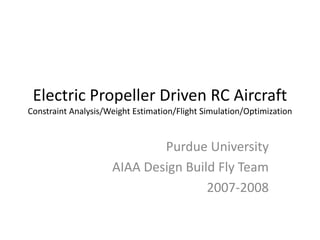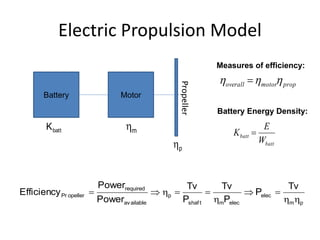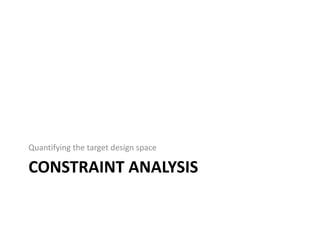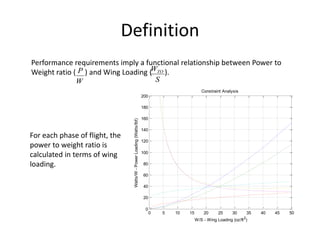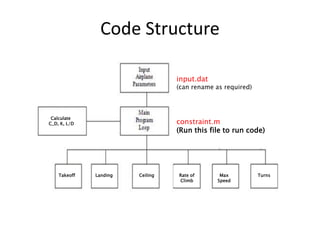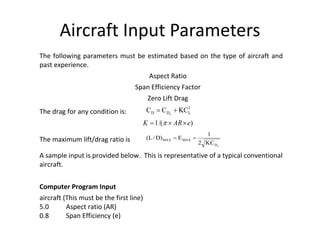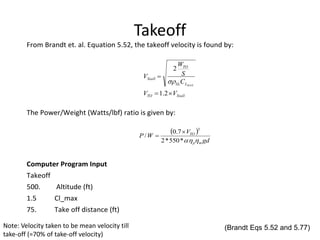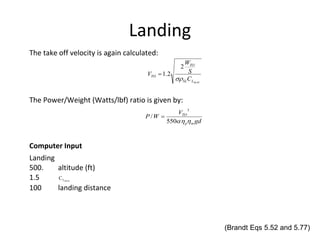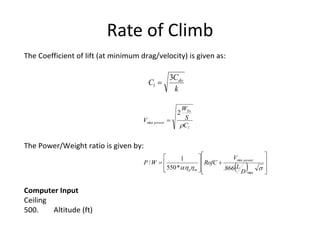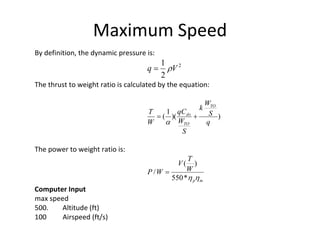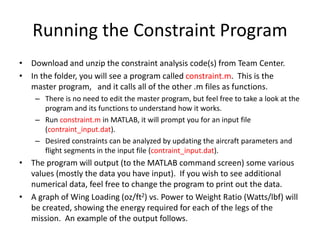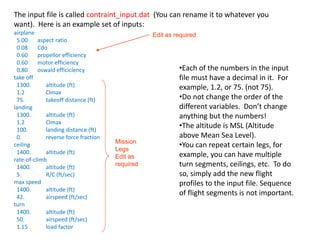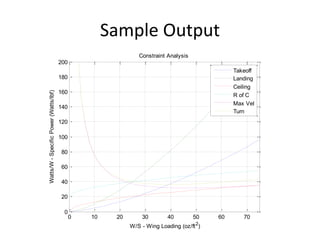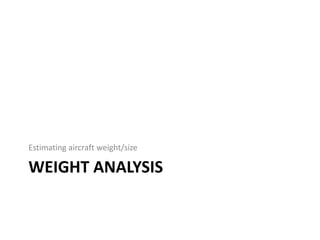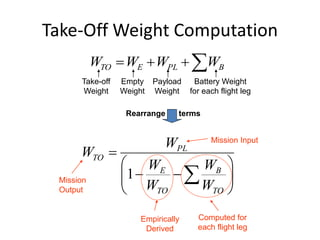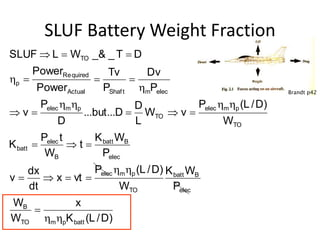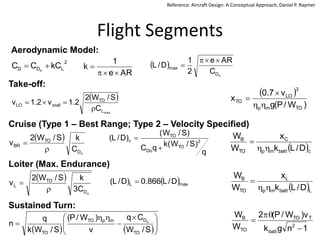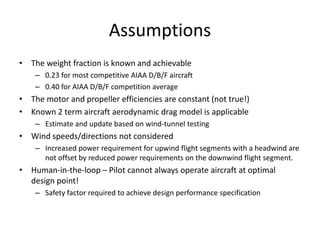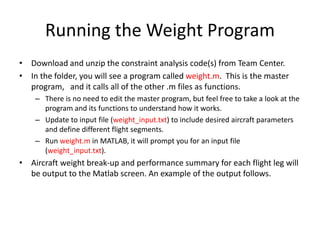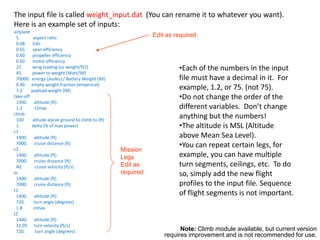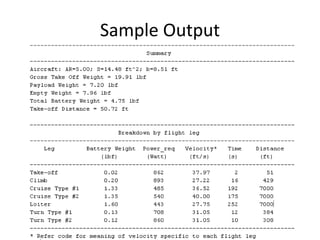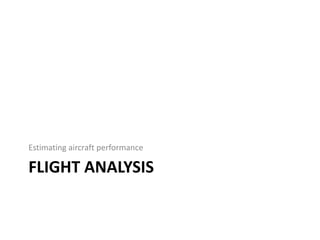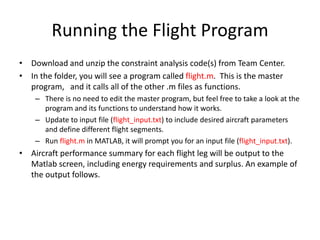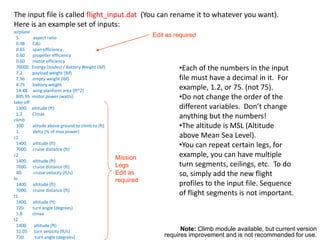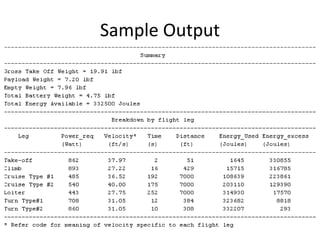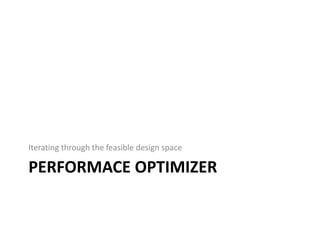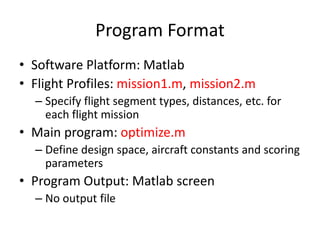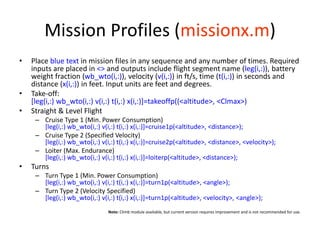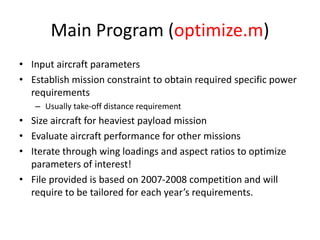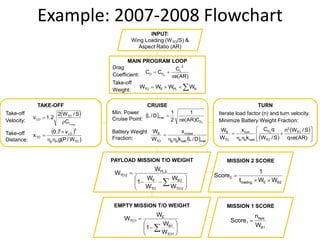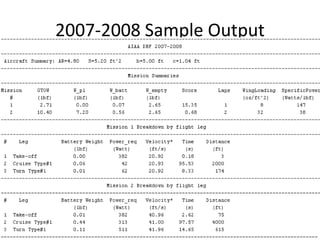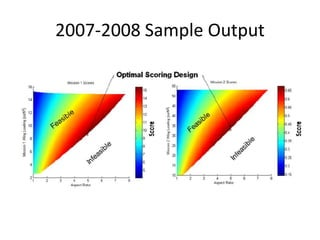1 sur 35
Publicité

### Electric_Propeller_Aircraft_Sizing.pptx

1. Electric Propeller Driven RC Aircraft Constraint Analysis/Weight Estimation/Flight Simulation/Optimization Purdue University AIAA Design Build Fly Team 2007-2008
2. Battery Motor Propeller m  p  p m elec elec m shaf t p available required opeller Pr Tv P P Tv P Tv Power Power Efficiency           batt batt W E K  prop motor overall     Electric Propulsion Model Measures of efficiency: batt K Battery Energy Density:
3. CONSTRAINT ANALYSIS Quantifying the target design space
4. Definition Performance requirements imply a functional relationship between Power to Weight ratio ( ) and Wing Loading ( ). W P S WTO 0 5 10 15 20 25 30 35 40 45 50 0 20 40 60 80 100 120 140 160 180 200 Constraint Analysis W/S - Wing Loading (oz/ft2 ) Watts/W - Power Loading (Watts/lbf) For each phase of flight, the power to weight ratio is calculated in terms of wing loading.
5. Code Structure input.dat (can rename as required) constraint.m (Run this file to run code) Turns Turns Max Speed Rate of Climb Ceiling Landing Takeoff Calculate C_D, K, L/D
6. Aircraft Input Parameters The following parameters must be estimated based on the type of aircraft and past experience. Aspect Ratio Span Efficiency Factor Zero Lift Drag The drag for any condition is: 2 L D D KC C C o   ) /( 1 e AR K     The maximum lift/drag ratio is o D MAX MAX KC 2 1 E ) D / L (   A sample input is provided below. This is representative of a typical conventional aircraft. Computer Program Input aircraft (This must be the first line) 5.0 Aspect ratio (AR) 0.8 Span Efficiency (e)
7. Takeoff From Brandt et. al. Equation 5.52, the takeoff velocity is found by: Stall TO L SL TO Stall V V C S W V MAX    2 . 1 2  The Power/Weight (Watts/lbf) ratio is given by:   gd V W P m p TO   * 550 * 2 7 . 0 / 3   Computer Program Input Takeoff 500. Altitude (ft) 1.5 Cl_max 75. Take off distance (ft) Note: Velocity taken to be mean velocity till take-off (=70% of take-off velocity) (Brandt Eqs 5.52 and 5.77)
8. Landing The take off velocity is again calculated: MAX L SL TO TO C S W V  2 2 . 1  The Power/Weight (Watts/lbf) ratio is given by: gd V W P m p TO   550 / 3  Computer Input Landing 500. altitude (ft) 1.5 MAX L C 100 landing distance (Brandt Eqs 5.52 and 5.77)
9. Ceiling The Coefficient of lift (at minimum drag/velocity) is given as: k C C do l 3  l To y C S W V  2  The Power/Weight ratio is given by:    g V W P m p y * 550 * 866 . /  Computer Input Ceiling 500. Altitude (ft)
10. Rate of Climb The Coefficient of lift (at minimum drag/velocity) is given as: k C C do l 3  l To power C S W V  2 min  The Power/Weight ratio is given by:                        max min 866 . * 550 1 / D L V RofC W P power m p Computer Input Ceiling 500. Altitude (ft)
11. Maximum Speed By definition, the dynamic pressure is: 2 2 1 V q   The thrust to weight ratio is calculated by the equation: ) )( 1 ( q S W k S W qC W T TO TO do    The power to weight ratio is: m p W T V W P   * 550 ) ( /  Computer Input max speed 500. Altitude (ft) 100 Airspeed (ft/s)
12. Turn The Power/Weight ratio for turns is determined the same way as that of the Maximum Speed function but with a load factor (dependent on bank angle) in the thrust-to-weight ratio equation. 2 2 1 V q   ) )( 1 ( 2 q S W k n S W qC W T To To do    m p W T V W P   * 550 /  Computer Input turn 35000. Altitude (ft) 660. airspeed (ft/sec) 1.15 load factor – n
13. Running the Constraint Program • Download and unzip the constraint analysis code(s) from Team Center. • In the folder, you will see a program called constraint.m. This is the master program, and it calls all of the other .m files as functions. – There is no need to edit the master program, but feel free to take a look at the program and its functions to understand how it works. – Run constraint.m in MATLAB, it will prompt you for an input file (contraint_input.dat). – Desired constraints can be analyzed by updating the aircraft parameters and flight segments in the input file (contraint_input.dat). • The program will output (to the MATLAB command screen) some various values (mostly the data you have input). If you wish to see additional numerical data, feel free to change the program to print out the data. • A graph of Wing Loading (oz/ft2) vs. Power to Weight Ratio (Watts/lbf) will be created, showing the energy required for each of the legs of the mission. An example of the output follows.
14. The input file is called contraint_input.dat (You can rename it to whatever you want). Here is an example set of inputs: airplane 5.00 aspect ratio 0.08 Cdo 0.60 propellor efficiency 0.60 motor efficiency 0.80 oswald efficiciency take off 1300. altitude (ft) 1.2 Clmax 75. takeoff distance (ft) landing 1300. altitude (ft) 1.2 Clmax 100. landing distance (ft) 0. reverse force fraction ceiling 1400. altitude (ft) rate-of-climb 1400. altitude (ft) 5. R/C (ft/sec) max speed 1400. altitude (ft) 42. airspeed (ft/sec) turn 1400. altitude (ft) 50. airspeed (ft/sec) 1.15 load factor •Each of the numbers in the input file must have a decimal in it. For example, 1.2, or 75. (not 75). •Do not change the order of the different variables. Don’t change anything but the numbers! •The altitude is MSL (Altitude above Mean Sea Level). •You can repeat certain legs, for example, you can have multiple turn segments, ceilings, etc. To do so, simply add the new flight profiles to the input file. Sequence of flight segments is not important. Mission Legs Edit as required Edit as required
15. Sample Output 0 10 20 30 40 50 60 70 0 20 40 60 80 100 120 140 160 180 200 Constraint Analysis W/S - Wing Loading (oz/ft2 ) Watts/W - Specific Power (Watts/lbf) Takeoff Landing Ceiling R of C Max Vel Turn
16. WEIGHT ANALYSIS Estimating aircraft weight/size
17. Rearrange terms             TO B TO E PL TO W W W W W W 1 Take-off Weight Empty Weight Payload Weight Battery Weight for each flight leg     B PL E TO W W W W Mission Input Empirically Derived Mission Output Computed for each flight leg Take-Off Weight Computation
18. SLUF Battery Weight Fraction ) D / L ( K x W W P W K W ) D / L ( P vt x dt dx v P W K t W t P K W ) D / L ( P v W L D D ... but ... D P v P Dv P Tv Power Power D T _ _& W L SLUF batt p m TO B elec B batt TO p m elec elec B batt B elec batt TO p m elec TO p m elec elec m Shaft Actual quired Re p TO                              Brandt p42
19. Flight Segments  c batt m p C TO B D / L k x W W      max L TO stall LO C S / W 2 2 . 1 v 2 . 1 v       ) W / P ( g v 7 . 0 x TO m p 3 LO TO       o D TO BR C k S / W 2 v   Take-off: Cruise (Type 1 – Best Range; Type 2 – Velocity Specified) Sustained Turn: 2 L D D kC C C o     o D max C AR e 2 1 D / L     Aerodynamic Model:  L batt m p L TO B D / L k x W W      o D TO L C 3 k S / W 2 v   Loiter (Max. Endurance)  max L D / L 866 . 0 ) D / L (                  S / W C q v ) W / P ( S / W k q n TO D m p TO TO o AR e 1 k     1 n g k v ) W / P ( 2 W W 2 batt T TO TO B    Reference: Aircraft Design: A Conceptual Approach, Daniel P. Raymer q ) S / W ( k q C ) S / W ( ) D / L ( 2 TO Do TO c  
20. Assumptions • The weight fraction is known and achievable – 0.23 for most competitive AIAA D/B/F aircraft – 0.40 for AIAA D/B/F competition average • The motor and propeller efficiencies are constant (not true!) • Known 2 term aircraft aerodynamic drag model is applicable – Estimate and update based on wind-tunnel testing • Wind speeds/directions not considered – Increased power requirement for upwind flight segments with a headwind are not offset by reduced power requirements on the downwind flight segment. • Human-in-the-loop – Pilot cannot always operate aircraft at optimal design point! – Safety factor required to achieve design performance specification
21. Running the Weight Program • Download and unzip the constraint analysis code(s) from Team Center. • In the folder, you will see a program called weight.m. This is the master program, and it calls all of the other .m files as functions. – There is no need to edit the master program, but feel free to take a look at the program and its functions to understand how it works. – Update to input file (weight_input.txt) to include desired aircraft parameters and define different flight segments. – Run weight.m in MATLAB, it will prompt you for an input file (weight_input.txt). • Aircraft weight break-up and performance summary for each flight leg will be output to the Matlab screen. An example of the output follows.
22. The input file is called weight_input.dat (You can rename it to whatever you want). Here is an example set of inputs: airplane 5. aspect ratio 0.08 Cdo 0.65 span efficiency 0.60 propeller efficiency 0.60 motor efficiency 22. wing loading (oz weight/ft2) 45. power to weight (Watt/lbf) 70000. energy (Joules) / Battery Weight (lbf) 0.40 empty weight fraction (emperical) 7.2 payload weight (lbf) take-off 1300. altitude (ft) 1.2 Clmax climb 100 alitude above ground to climb to (ft) 1. delta (% of max power) c1 1400. altitude (ft) 7000. cruise distance (ft) c2 1400. altitude (ft) 7000. cruise distance (ft) 40. cruise velocity (ft/s) lo 1400. altitude (ft) 7000. cruise distance (ft) t1 1400. altitude (ft) 720. turn angle (degrees) 1.8 clmax t2 1400. altitude (ft) 31.05 turn velocity (ft/s) 720. turn angle (degrees) •Each of the numbers in the input file must have a decimal in it. For example, 1.2, or 75. (not 75). •Do not change the order of the different variables. Don’t change anything but the numbers! •The altitude is MSL (Altitude above Mean Sea Level). •You can repeat certain legs, for example, you can have multiple turn segments, ceilings, etc. To do so, simply add the new flight profiles to the input file. Sequence of flight segments is not important. Mission Legs Edit as required Edit as required Note: Climb module available, but current version requires improvement and is not recommended for use.
23. Sample Output
24. FLIGHT ANALYSIS Estimating aircraft performance
25. Running the Flight Program • Download and unzip the constraint analysis code(s) from Team Center. • In the folder, you will see a program called flight.m. This is the master program, and it calls all of the other .m files as functions. – There is no need to edit the master program, but feel free to take a look at the program and its functions to understand how it works. – Update to input file (flight_input.txt) to include desired aircraft parameters and define different flight segments. – Run flight.m in MATLAB, it will prompt you for an input file (flight_input.txt). • Aircraft performance summary for each flight leg will be output to the Matlab screen, including energy requirements and surplus. An example of the output follows.
26. The input file is called flight_input.dat (You can rename it to whatever you want). Here is an example set of inputs: airplane 5. aspect ratio 0.08 Cdo 0.65 span efficiency 0.60 propeller efficiency 0.60 motor efficiency 70000. Energy (Joules) / Battery Weight (lbf) 7.2 payload weight (lbf) 7.96 empty weight (lbf) 4.75 battery weight 14.48 wing planform area (ft^2) 895.95 motor power (watts) take-off 1300. altitude (ft) 1.2 Clmax climb 100 alitude above ground to climb to (ft) 1. delta (% of max power) c1 1400. altitude (ft) 7000. cruise distance (ft) c2 1400. altitude (ft) 7000. cruise distance (ft) 40. cruise velocity (ft/s) lo 1400. altitude (ft) 7000. cruise distance (ft) t1 1400. altitude (ft) 720. turn angle (degrees) 1.8 clmax t2 1400. altitude (ft) 31.05 turn velocity (ft/s) 720. turn angle (degrees) •Each of the numbers in the input file must have a decimal in it. For example, 1.2, or 75. (not 75). •Do not change the order of the different variables. Don’t change anything but the numbers! •The altitude is MSL (Altitude above Mean Sea Level). •You can repeat certain legs, for example, you can have multiple turn segments, ceilings, etc. To do so, simply add the new flight profiles to the input file. Sequence of flight segments is not important. Mission Legs Edit as required Edit as required Note: Climb module available, but current version requires improvement and is not recommended for use.
27. Sample Output
28. PERFORMACE OPTIMIZER Iterating through the feasible design space
29. Program Format • Software Platform: Matlab • Flight Profiles: mission1.m, mission2.m – Specify flight segment types, distances, etc. for each flight mission • Main program: optimize.m – Define design space, aircraft constants and scoring parameters • Program Output: Matlab screen – No output file
30. Mission Profiles (missionx.m) • Place blue text in mission files in any sequence and any number of times. Required inputs are placed in <> and outputs include flight segment name (leg(i,:)), battery weight fraction (wb_wto(i,:)), velocity (v(i,:)) in ft/s, time (t(i,:)) in seconds and distance (x(i,:)) in feet. Input units are feet and degrees. • Take-off: [leg(i,:) wb_wto(i,:) v(i,:) t(i,:) x(i,:)]=takeoffp((<altitude>, <Clmax>) • Straight & Level Flight – Cruise Type 1 (Min. Power Consumption) [leg(i,:) wb_wto(i,:) v(i,:) t(i,:) x(i,:)]=cruise1p(<altitude>, <distance>); – Cruise Type 2 (Specified Velocity) [leg(i,:) wb_wto(i,:) v(i,:) t(i,:) x(i,:)]=cruise2p(<altitude>, <distance>, <velocity>); – Loiter (Max. Endurance) [leg(i,:) wb_wto(i,:) v(i,:) t(i,:) x(i,:)]=loiterp(<altitude>, <distance>); • Turns – Turn Type 1 (Min. Power Consumption) [leg(i,:) wb_wto(i,:) v(i,:) t(i,:) x(i,:)]=turn1p(<altitude>, <angle>); – Turn Type 2 (Velocity Specified) [leg(i,:) wb_wto(i,:) v(i,:) t(i,:) x(i,:)]=turn1p(<altitude>, <velocity>, <angle>); Note: Climb module available, but current version requires improvement and is not recommended for use.
31. Main Program (optimize.m) • Input aircraft parameters • Establish mission constraint to obtain required specific power requirements – Usually take-off distance requirement • Size aircraft for heaviest payload mission • Evaluate aircraft performance for other missions • Iterate through wing loadings and aspect ratios to optimize parameters of interest! • File provided is based on 2007-2008 competition and will require to be tailored for each year’s requirements.
32. Example: 2007-2008 Flowchart INPUT: Wing Loading (WTO/S) & Aspect Ratio (AR) MAIN PROGRAM LOOP Drag Coefficient: Take-off Weight: TAKE-OFF Take-off Velocity: Take-off Distance: PAYLOAD MISSION T/O WEIGHT             2 TO 2 B TO E 2 PL 2 TO W W W W 1 W W     B PL E TO W W W W ) AR ( e C C C 2 L D D o      max L TO LO C S / W 2 2 . 1 v     ) W / P ( g v 7 . 0 x TO m p 3 LO TO     CRUISE Min. Power Cruise Point: Battery Weight Fraction: TURN Iterate load factor (n) and turn velocity. Minimize Battery Weight Fraction:  max batt p p cruise TO B D / L k x W W                   ) AR ( e q S / W n S / W q C k x W W TO 2 TO D batt p p turn TO B o   o D max C ) AR ( e 1 2 1 D / L   EMPTY MISSION T/O WEIGHT            1 TO 1 B E 1 TO W W 1 W W MISSION 2 SCORE MISSION 1 SCORE 2 B E loading 2 W W t 1 Score    1 B laps 1 W n Score 
33. 2007-2008 Sample Output
34. 2007-2008 Sample Output
35. Contacts Pritesh Mody (pcmody@purdue.edu) Kyle Noth (knoth@purdue.edu)
Publicité